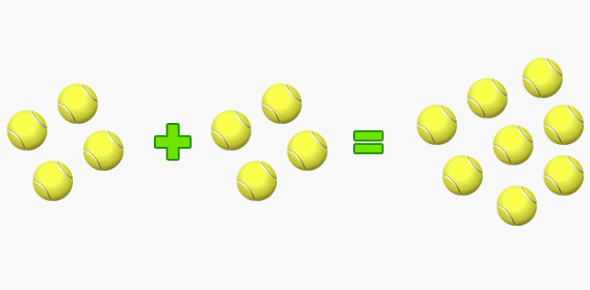# Maths Quiz About Doubling And Multiplication

20 Questions | Total Attempts: 72SettingsThis is a simple maths quiz for kids. Take on this quiz as a small test to understand the basic counting and concepts of maths. Multiplication by 2 or doubling is a simple yet integral part of mathematical operations. All the best for the quiz.

• 1.
6+6=
• 2.
5+6=
• 3.
8+8=
• 4.
6+7=
• 5.
9+9=
• 6.
4+5=
• 7.
7+7=
• 8.
2+3=
• 9.
9+10=
• 10.
7+6=
• 11.
8+9=
• 12.
5+4=
• 13.
6+5=
• 14.
2+3=
• 15.
7+8=
• 16.
4+3=
• 17.
5+5=
• 18.
3+3=
• 19.
8+7=
• 20.
4+4=
Related Topics